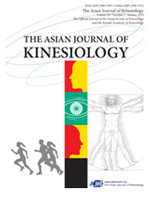상세검색
최근 검색어 전체 삭제
다국어입력
즐겨찾기0KCI등재 학술저널

# Utilization of the Speed&#8211;Effectiveness Index for Evaluating the Jump Performance of Female College Students in a Running-Long Jump PE Class

• 등재여부 : KCI등재
• 2019.04
• 1 - 7 (7 pages)

OBJECTIVES This study investigated the speed&#8211;effectiveness index (SEI) profile for running long jump in female college students and its applicability in evaluating jump skill. METHODS Sixty-two female college students were chosen as subjects. First, a multiple regression analysis was performed by setting jump distance as the dependent variable and height, weight, and 50-meter run time as independent variables. The SEI was calculated as the ratio between the actual jump distance and estimated jump distance derived by substituting the subject’s 50-meter run time into a liner regression equation correlating 50-meter run time with an actual jump distance (based on all subjects). RESULTS The only significant regression coefficient was the 50-meter run (p < .05), which accounted for 59.5% of jump distance variance. The SEI, which refers to the effective (or ineffective) use of speed in jumping distance, is considered a normally distributed jump skill metric. Furthermore, no significant differences in physique were found between high-SEI (greater than or equal to 1) and low-SEI (below 1) groups. CONCLUSIONS The SEI could provide a relative evaluation of jumping performance depending on physical resources without consideration of physique.

Introduction

Methods

Results

Discussion

Conclusions

Conflicts of Interest

References

로딩중